A gift box has dimensions of: 8 1/2 inches, 5 1/2 inches, and 2 1/2 inches, respectively. How many cubes with side lengths of 1/2 inches would be needed to fill the gift box?

n =  935

### Step-by-step explanation: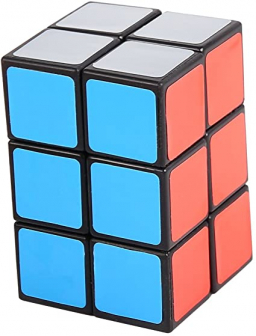Did you find an error or inaccuracy? Feel free to write us. Thank you!Tips to related online calculators
Tip: Our volume units converter will help you with the conversion of volume units.

## Related math problems and questions:

• A box 2A box has a length of 4 1/2 inches, a width of 3 2/3 inches and a height of 8 1/4 inches. What is the volume of the box?
• Cubes into cuboidHow many 12 centimeter cubes fit into the block (cuboid) with 6dm, 8.4dm, and 4.8?
• Identical cubesFrom the smallest number of identical cubes whose edge length is expressed by a natural number, can we build a block with dimensions 12dm x 16dm x 20dm?
• Into boxHow many cubes with an edge of 2.5 cm fit into a box measuring 11.6 cm; 8.9 cm and 13.75 cm?
• Jewelry box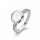The bottom of Zeyda's jewelry box is a rectangle with the length of 5 3/8 inches and a width of 3 1/4 inches. What is the area of the bottom of the jewelry box?
• Ribbon on the cube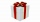A cubical gift box is tied with a piece of ribbon. If the total length of the free ends and the bow is 18 inches, what is the length of the ribbon used? (Each side of the cube is 6 inches).
• Roll of wallpaperAn art student uses a roll of wallpaper to decorate two gift boxes. The student will use 3 1/3 yards of paper for one box and 5/6 yard of paper for the other box. The paper must be cut into pieces that are 1/6 yard long. How many pieces will the student c
• Hole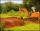They fill the shape hole with dimensions 2.9 m, 17 m, 15.2 m with 97 m3 of soil. How many percent does it fill up?
• Cubic inches to cups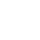5-3/8" x 4" x 2-5/8" container. How many equals cups?
• TetrapackHow high should be the milk box in the shape of a prism with base dimensions 8 cm and 8.8 cm if its volume is 1 liter?
• The woodenThe wooden block measures 12 cm, 24 cm, and 30 cm. Peter wants to cut it into several identical cubes. At least how many cubes can he get?
• Lin hasLin has a cake pan that has a length of 8/3 inches, a width of 15/4 inches and a height of 3/2 inches. What is the volume of the cake pan?
• Building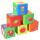Lenka has 22 cubes for the construction of building comprising three cubes in height, the width of two cubes, and the length of four cubes. Is she able to build an building with these cubes?
• Dana helped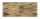Dana helped her dad build a sandbox for her younger sister. The sandbox is shaped like a rectangular prism that is 4 1/2 feet long and 4 feet wide. Dana used bags of sand to fill the sandbox 1/2 of a foot deep. Each bag contained 1/2 of a cubic foot of sa
• Wooden box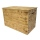The block-shaped box was placed on the ground, leaving a rectangular print with 3 m and 2 m. When flipped over to another wall, a print with dimensions of 0.5 m and 3 m remained in the sand. What is the volume of the wooden box?
• Concrete boxThe concrete box with walls thick 7 cm has the following external dimensions: length 1.8 m, width 44 cm and height 46 cm. How many liters of soil can fit if I fill it to the brim?
• Milk cartonsHow much paper do we need for 12 tetra packs with dimensions 6 cm, 11 cm, and 20 cm? Will 1 liter of milk fit in the box?